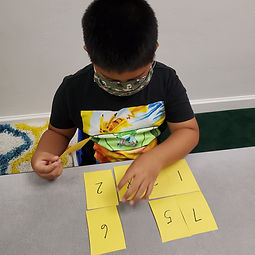## Ms. Kylie

### Target 1​

###### Lesson Type:

Continuation

Number Operation

:

Computation

Solve multi-step word problems.

###### 1:

Identify the correct arithmetic process based on the information presented in word problems.

###### 2:

Find the key words in word problems that indicate the correct arithmetic process to use.

###### 3:

Find and use the needed information in a word problem to solve.

5th

###### Vocabulary:

CUBES, Operational words

Activities:

-Students were given multi-step word problems to solve using CUBES to identify the correct operations and information needed

-Students wrote an equation and solved### Home Exploration

###### Guiding Questions:## Absent Students:

### Target 2

:

###### 1:

Use ratio vocabulary to describe the relationships between two quantities.

###### 2:

Understand that a ratio expresses the comparison between two quantities.

6th

###### Vocabulary:

Ratio, Comparison

Activities:

-Students were given several pictures of groups items/things and wrote a ratio for each picture. They labled their ratios using word form and the semi colon### Home Exploration

###### Guiding Questions:### Target 3

:

###### 1:

Use the given information in a problem to identify the correct answer.

###### 2:

Use the given information to determine which is not the correct answer.

###### 3:

Think outside of the box to solve problems.

###### 4:

Follow given rules to solve problems.

4th

###### Vocabulary:

Logic reasoning, Consecutive

Activities:

-Students use numbers 1-8 to find a way to arrange them in a 4x2 rectangle in which consecutive numbers were not allowed to touch on the sides or at the corners### Home Exploration